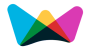#HELOC (Home Equity Line of Credit) Payment Calculation#HELOC Calculator

Instantly calculates your monthly HELOC payments (both Principal and Interest).

Amortization Schedule### HELOC Payment Calculator

Determine home equity loan payments with our calculator.

## Home Equity Line of Credit(HELOC) Formulas

Monthly Interest Only Payment = CHB × RATE

Monthly Principal & Interest Payment = (CHB × RATE) × ( (1 + RATE) (12 × RP) ) / ( (1 + RATE) (12 × RP) - 1 )

Where,

CHB is Current HELOC Balance

RP is Repayment Period (years)

RATE(monthly interest rate) = Decimal Rate / 12 , or RATE = (Annual Interest Rate / 100) / 12

###More Calculators ►

Weekly Interest Calculator

Short Term Interest Calculator

Savings Interest Calculator